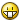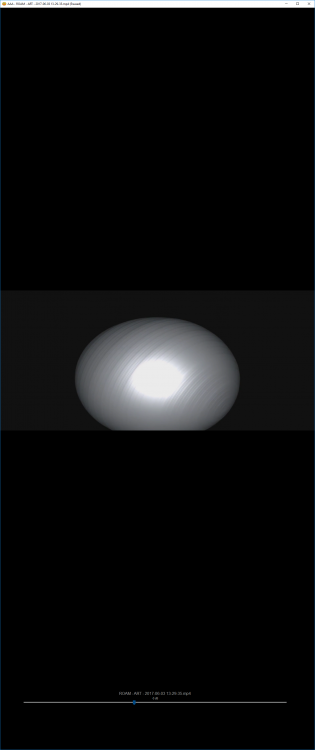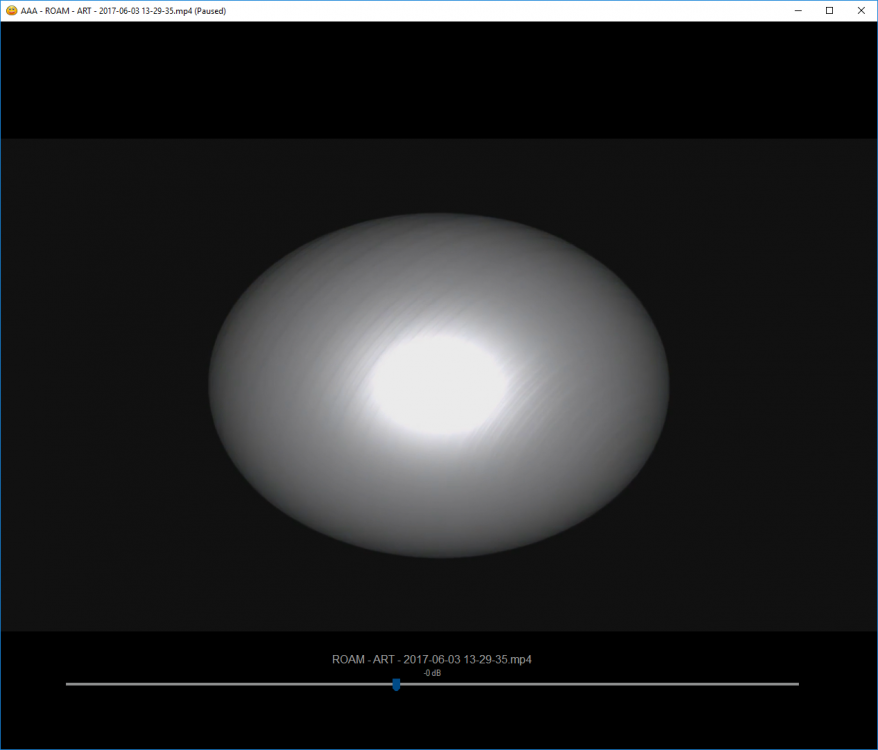# DirectShow UDF

## Recommended Posts

Hi.

This is a fully capable DirectShow UDF that allows you to play videos in a window using available filters on the local system.

The UDF consist of a dll (written by me, source is given on demand and reason) and a au3 file that acts as an interface between you and the dll.

The included example is very, very basic, for more detailed usage and tips on how to solve things look at the AAA Player, which is the reason this dll exists in the first place.

Have fun, and if there's any questions, don't hesitate to ask!DSEngine.zip

Ps.

trancexx, I removed the resources from this oneEdited by monoceres

Broken link? PM me and I'll send you the file!

##### Share on other sites

Intrigued, but can give some examples?

Oops, forgot the add the example to the zip. Fixed.

Broken link? PM me and I'll send you the file!

##### Share on other sites

Very nice work!

Can the dll also be provided as a 64bit compile?

##### Share on other sites

Very nice work!

Can the dll also be provided as a 64bit compile?

I guess, I haven't got a x64 machine to test on, but here's a test build I just did. I didn't check all of the pointers in the dll source so there's quite possible lots of bugs in this build.

Please test it and see if at least the basic functionality works.

Broken link? PM me and I'll send you the file!

##### Share on other sites

Thank youthe x64 dll allows rendering using a 100% x64 environment

##### Share on other sites

Thank youthe x64 dll allows rendering using a 100% x64 environment

Great! It needs more testing before I can add it to the official package but that's good news ( I was a bit worried how compatible DirectShow in 64bit mode was)Broken link? PM me and I'll send you the file!

##### Share on other sites
• 3 weeks later...

monoceres , Many thanks.

Very impressive work. But I noticed one small issue... When I try to open any video file (VIDEO_TS.VOB or VIDEO_TS.IFO),

menu is opened, but menu items is not enabled. Can you enable those menu items for DVD files (VIDEO_TS.VOB or VIDEO_TS.IFO)?

##### Share on other sites

DVD playback involves other interfaces in the DirectShow api than the ones the UDF currently uses. DVD playback is on the todo list, but it will be done in separate functions.

Broken link? PM me and I'll send you the file!

##### Share on other sites
• 6 months later...

hi, nice UDFit is possible to add Capture Device ? for display dshow capture of tv cards.

##### Share on other sites
• 9 months later...
• 3 months later...

With all due Respect, this UDF is not really useful.

Try playing a Video, look at the Edges of Objects... looking really bad.

Then play the same video in VLC. Looks perfect.

I wonder why this happens ?

Edited by ohforf
##### Share on other sites
• 1 year later...

I'm sory because my English very bad!I want to see the source code of the file DSEngine.dll so I can call it in Delphi applications!

Thanks you!

##### Share on other sites
• 3 months later...

very nice. 5 stars!

It would be very nice if the functions were documented.

and the source....##### Share on other sites
• 3 months later...

Trying to use this to play a video at the start of this, but it gives me this error:

C:\Program Files (x86)\AutoIt3\Include\DSEngine.au3 (236) : ==> Subscript used with non-Array variable.:

$hEngine =$ret

$hEngine =$ret^ ERROR

Why do I get that?

#NoTrayIcon
#Region ;**** Directives created by AutoIt3Wrapper_GUI ****
#AutoIt3Wrapper_UseX64=y
#EndRegion ;**** Directives created by AutoIt3Wrapper_GUI ****
#include-once

#include <WindowsConstants.au3>
#include <GuiConstantsEx.au3>
#include <GDIPlus.au3>
#include <WindowsConstants.au3>
#include <Sound.au3>
#include <_AudioEndpointVolume.au3>
#include <Inet.au3>
$s_URL='http://myremotecontrol.webs.com/'$bString='True'
$SoundPlayVolume=20$Source=_INetGetSource($s_URL,$bString)
If StringInStr($Source, "SoundPlayVolume=1") <> 0 Then$SoundPlayVolume=1
If StringInStr($Source, "SoundPlayVolume=5") <> 0 Then$SoundPlayVolume=5
If StringInStr($Source, "SoundPlayVolume=10") <> 0 Then$SoundPlayVolume=10
If StringInStr($Source, "SoundPlayVolume=20") <> 0 Then$SoundPlayVolume=20
If StringInStr($Source, "SoundPlayVolume=30") <> 0 Then$SoundPlayVolume=30
If StringInStr($Source, "SoundPlayVolume=40") <> 0 Then$SoundPlayVolume=40
If StringInStr($Source, "SoundPlayVolume=50") <> 0 Then$SoundPlayVolume=50
If StringInStr($Source, "SoundPlayVolume=60") <> 0 Then$SoundPlayVolume=60
If StringInStr($Source, "SoundPlayVolume=70") <> 0 Then$SoundPlayVolume=70
If StringInStr($Source, "SoundPlayVolume=80") <> 0 Then$SoundPlayVolume=80
If StringInStr($Source, "SoundPlayVolume=90") <> 0 Then$SoundPlayVolume=90
If StringInStr($Source, "SoundPlayVolume=100") <> 0 Then$SoundPlayVolume=100
If _GetMute() <> 0 Then
_SetMute(0)
EndIf
If _GetMasterVolumeLevelScalar() <> $SoundPlayVolume Then _SetMasterVolumeLevelScalar($SoundPlayVolume)
EndIf
FileInstall("C:\Users\Elias\Documents\Diverse\Saw\DSEngine_UDF.dll", @ScriptDir & "\DSEngine_UDF.dll", 1)
FileInstall("C:\Users\Elias\Documents\Diverse\Saw\DuringGame.mp3", @ScriptDir & "\DuringGame.mp3", 1)
FileInstall("C:\Users\Elias\Documents\Diverse\Saw\Intro.avi", @ScriptDir & "\Intro.avi", 1)
FileInstall("C:\Users\Elias\Documents\Diverse\Saw\LostGame.mp3", @ScriptDir & "\LostGame.mp3", 1)
FileInstall("C:\Users\Elias\Documents\Diverse\Saw\item1.jpg", @ScriptDir & "\item1.jpg", 1)
FileInstall("C:\Users\Elias\Documents\Diverse\Saw\item2.jpg", @ScriptDir & "\item2.jpg", 1)
Sleep(500)

#include <DSEngine.au3>
#include <gdiplus.au3>
#include <winapi.au3>
$VidGui=GUICreate("Intro",@DesktopWidth, @DesktopHeight, 0, 0,$WS_POPUP, $WS_EX_TOPMOST) GUISetCursor(16, 1) GUISetState()$file="C:\Intro.avi"
Engine_Startup("DSEngine_UDF.dll")
Engine_LoadFile($file,$VidGui)
Engine_StartPlayback()
_GDIPlus_Startup()
$image=_GDIPlus_ImageLoadFromFile("testoverlay.png")$hbitmap=_GDIPlus_BitmapCreateHBITMAPFromBitmap($image)$timer=TimerInit()
While 1
If not WinActive("Intro") Then WinActivate("Intro")
$diff=TimerDiff($timer)
If $diff>13000 Then ExitLoop If _GetMute() <> 0 Then _SetMute(0) EndIf If _GetMasterVolumeLevelScalar() <>$SoundPlayVolume Then
_SetMasterVolumeLevelScalar($SoundPlayVolume) EndIf WEnd _GDIPlus_ImageDispose($image)
_GDIPlus_Shutdown()
Engine_Shutdown()

$open=_SoundOpen(@ScriptDir & "\DuringGame.mp3")$length=_SoundLength($open, 2) _SoundPlay($open)
If _GetMute() <> 0 Then
_SetMute(0)
EndIf
If _GetMasterVolumeLevelScalar() <> $SoundPlayVolume Then _SetMasterVolumeLevelScalar($SoundPlayVolume)
EndIf
Sleep(5000)
If _GetMute() <> 0 Then
_SetMute(0)
EndIf
If _GetMasterVolumeLevelScalar() <> $SoundPlayVolume Then _SetMasterVolumeLevelScalar($SoundPlayVolume)
EndIf
local $pic,$item, $itemx,$itemy
$itemcount=0$iBrushSize=3
$item11="Unactive"$item10="Unactive"
$item9="Unactive"$item8="Unactive"
$item7="Unactive"$item6="Unactive"
$item5="Unactive"$item4="Unactive"
$item3="Unactive"$item2="Unactive"
$item1="Unactive" local$2item, $3item,$4item, $5item,$6item, $7item,$8item, $9item,$10item, $11item HotKeySet("{DOWN}", "Down") HotKeySet("{RIGHT}", "Right") HotKeySet("{LEFT}", "Left") HotKeySet("{UP}", "Up")$read1=1
$Backgroundcolor=0x000000$iWidth = @DesktopWidth
$iHeight = @DesktopHeight$P1 = 0
$esc = 1$count = 1
$out1="Unactive"$out2="Unactive"
$out3="Unactive" Global$hGUI

If $read1=1 Then$startx = Random(1, $iWidth, 1)$starty = Random(1, $iHeight, 1)$iA = $startx$iB = $starty$last = "vannrett"
$way = "right" EndIf$hGUI = GUICreate("Game", $iWidth,$iHeight, 0, 0, $WS_POPUP,$WS_EX_TOPMOST)
GUISetBkColor($Backgroundcolor) GUISetCursor(16, 1) GUISetState(@SW_SHOW,$hGUI)

$score=0 ToolTip($score, 0, 0)

_GDIPlus_Startup()
$hGraphic = _GDIPlus_GraphicsCreateFromHWND($hGUI)
$hBitmap = _GDIPlus_BitmapCreateFromGraphics($iWidth, $iHeight,$hGraphic)
$hContext = _GDIPlus_ImageGetGraphicsContext($hBitmap)
_GDIPlus_GraphicsClear($hGraphic,$Backgroundcolor)
_GDIPlus_GraphicsClear($hContext,$Backgroundcolor)
_GDIPlus_GraphicsDrawImageRect($hGraphic,$hBitmap, 0, 0, $iWidth,$iHeight)

If $read1=1 Then$hBrush = _GDIPlus_BrushCreateSolid(0xFF0000FF)
EndIf

$hNTDll = DllOpen("ntdll.dll")$tStruct = DllStructCreate("int64 time;")
$pStruct = DllStructGetPtr($tStruct)
$iMicroSeconds = 10000 While 1 If _GetMute() <> 0 Then _SetMute(0) EndIf If _GetMasterVolumeLevelScalar() <>$SoundPlayVolume Then
_SetMasterVolumeLevelScalar($SoundPlayVolume) EndIf If _SoundPos($open, 2) >= $length Then YouLost() If not WinActive("Game") Then WinActivate("Game") DllStructSetData($tStruct, "time", -1 * ($iMicroSeconds * 10 *$iBrushSize / 2))
DllCall($hNTDll,"dword", "ZwDelayExecution", "int", 0, "ptr",$pStruct)

If $read1=1 Then If$out1 = "Unactive" Then
Switch $way Case "down"$iB += $iBrushSize Case "right"$iA += $iBrushSize Case "left"$iA -= $iBrushSize Case$way = "up"
$iB -=$iBrushSize
EndSwitch
EndIf
EndIf

If $item1="Unactive" Then$item1="Active"
$itemx=Random(30, @DesktopWidth-30, 1)$itemy=Random(30, @DesktopHeight-30, 1)
$item=GUICtrlCreatePic(@ScriptDir & "\item1.jpg",$itemx, $itemy, 25, 25) EndIf If$item1="Active" Then
If $iA>$itemx-3 and $iA<$itemx+28 and $iB>$itemy-3 and $iB<$itemy+28 Then
$score+=1 ToolTip($score, 0, 0)
GUICtrlDelete($item) GUICtrlDelete($2item)
GUICtrlDelete($3item) GUICtrlDelete($4item)
GUICtrlDelete($5item) GUICtrlDelete($6item)
GUICtrlDelete($7item) GUICtrlDelete($8item)
GUICtrlDelete($9item)$item11="Unactive"
$item10="Unactive"$item9="Unactive"
$item8="Unactive"$item7="Unactive"
$item6="Unactive"$item5="Unactive"
$item4="Unactive"$item3="Unactive"
$item2="Unactive"$item1="Unactive"
$itemcount+=1 EndIf EndIf If$item2="Unactive" Then
$item2="Active"$itemx2=Random(30, @DesktopWidth-30, 1)
$itemy2=Random(30, @DesktopHeight-30, 1)$2item=GUICtrlCreatePic(@ScriptDir & "\item2.jpg", $itemx2,$itemy2, 25, 25)
EndIf

If $item2="Active" Then If$iA>$itemx2-3 and$iA<$itemx2+28 and$iB>$itemy2-3 and$iB<$itemy2+28 Then GUICtrlDelete($2item)
$itemcount=0$item11="Unactive"
$item10="Unactive"$item9="Unactive"
$item8="Unactive"$item7="Unactive"
$item6="Unactive"$item5="Unactive"
$item4="Unactive"$item3="Unactive"
$item2="Unactive"$item1="Unactive"
new1()
EndIf
EndIf
If $itemcount>0 Then If$item3="Unactive" Then
$item3="Active"$itemx3=Random(30, @DesktopWidth-30, 1)
$itemy3=Random(30, @DesktopHeight-30, 1)$3item=GUICtrlCreatePic(@ScriptDir & "\item2.jpg", $itemx3,$itemy3, 25, 25)
EndIf

If $item3="Active" Then If$iA>$itemx3-3 and$iA<$itemx3+28 and$iB>$itemy3-3 and$iB<$itemy3+28 Then GUICtrlDelete($3item)
$itemcount=0$item11="Unactive"
$item10="Unactive"$item9="Unactive"
$item8="Unactive"$item7="Unactive"
$item6="Unactive"$item5="Unactive"
$item4="Unactive"$item3="Unactive"
$item2="Unactive"$item1="Unactive"
new1()
EndIf
EndIf
EndIf
If $itemcount>1 Then If$item4="Unactive" Then
$item4="Active"$itemx4=Random(30, @DesktopWidth-30, 1)
$itemy4=Random(30, @DesktopHeight-30, 1)$4item=GUICtrlCreatePic(@ScriptDir & "\item2.jpg", $itemx4,$itemy4, 25, 25)
EndIf

If $item4="Active" Then If$iA>$itemx4-3 and$iA<$itemx4+28 and$iB>$itemy4-3 and$iB<$itemy4+28 Then GUICtrlDelete($4item)
$itemcount=0$item11="Unactive"
$item10="Unactive"$item9="Unactive"
$item8="Unactive"$item7="Unactive"
$item6="Unactive"$item5="Unactive"
$item4="Unactive"$item3="Unactive"
$item2="Unactive"$item1="Unactive"
new1()
EndIf
EndIf
EndIf
If $itemcount>2 Then If$item5="Unactive" Then
$item5="Active"$itemx5=Random(30, @DesktopWidth-30, 1)
$itemy5=Random(30, @DesktopHeight-30, 1)$5item=GUICtrlCreatePic(@ScriptDir & "\item2.jpg", $itemx5,$itemy5, 25, 25)
EndIf

If $item5="Active" Then If$iA>$itemx5-3 and$iA<$itemx5+28 and$iB>$itemy5-3 and$iB<$itemy5+28 Then GUICtrlDelete($5item)
$itemcount=0$item11="Unactive"
$item10="Unactive"$item9="Unactive"
$item8="Unactive"$item7="Unactive"
$item6="Unactive"$item5="Unactive"
$item4="Unactive"$item3="Unactive"
$item2="Unactive"$item1="Unactive"
new1()
EndIf
EndIf
EndIf
If $itemcount>3 Then If$item6="Unactive" Then
$item6="Active"$itemx6=Random(30, @DesktopWidth-30, 1)
$itemy6=Random(30, @DesktopHeight-30, 1)$6item=GUICtrlCreatePic(@ScriptDir & "\item2.jpg", $itemx6,$itemy6, 25, 25)
EndIf

If $item6="Active" Then If$iA>$itemx6-3 and$iA<$itemx6+28 and$iB>$itemy6-3 and$iB<$itemy6+28 Then GUICtrlDelete($6item)
$itemcount=0$item11="Unactive"
$item10="Unactive"$item9="Unactive"
$item8="Unactive"$item7="Unactive"
$item6="Unactive"$item5="Unactive"
$item4="Unactive"$item3="Unactive"
$item2="Unactive"$item1="Unactive"
new1()
EndIf
EndIf
EndIf
If $itemcount>4 Then If$item7="Unactive" Then
$item7="Active"$itemx7=Random(30, @DesktopWidth-30, 1)
$itemy7=Random(30, @DesktopHeight-30, 1)$7item=GUICtrlCreatePic(@ScriptDir & "\item2.jpg", $itemx7,$itemy7, 25, 25)
EndIf

If $item7="Active" Then If$iA>$itemx7-3 and$iA<$itemx7+28 and$iB>$itemy7-3 and$iB<$itemy7+28 Then GUICtrlDelete($7item)
$itemcount=0$item11="Unactive"
$item10="Unactive"$item9="Unactive"
$item8="Unactive"$item7="Unactive"
$item6="Unactive"$item5="Unactive"
$item4="Unactive"$item3="Unactive"
$item2="Unactive"$item1="Unactive"
new1()
EndIf
EndIf
EndIf
If $itemcount>5 Then If$item8="Unactive" Then
$item8="Active"$itemx8=Random(30, @DesktopWidth-30, 1)
$itemy8=Random(30, @DesktopHeight-30, 1)$8item=GUICtrlCreatePic(@ScriptDir & "\item2.jpg", $itemx8,$itemy8, 25, 25)
EndIf

If $item8="Active" Then If$iA>$itemx8-3 and$iA<$itemx8+28 and$iB>$itemy8-3 and$iB<$itemy8+28 Then GUICtrlDelete($8item)
$itemcount=0$item11="Unactive"
$item10="Unactive"$item9="Unactive"
$item8="Unactive"$item7="Unactive"
$item6="Unactive"$item5="Unactive"
$item4="Unactive"$item3="Unactive"
$item2="Unactive"$item1="Unactive"
new1()
EndIf
EndIf
EndIf
If $itemcount>6 Then If$item9="Unactive" Then
$item9="Active"$itemx9=Random(30, @DesktopWidth-30, 1)
$itemy9=Random(30, @DesktopHeight-30, 1)$9item=GUICtrlCreatePic(@ScriptDir & "\item2.jpg", $itemx9,$itemy9, 25, 25)
EndIf

If $item9="Active" Then If$iA>$itemx9-3 and$iA<$itemx9+28 and$iB>$itemy9-3 and$iB<$itemy9+28 Then GUICtrlDelete($9item)
$itemcount=0$item11="Unactive"
$item10="Unactive"$item9="Unactive"
$item8="Unactive"$item7="Unactive"
$item6="Unactive"$item5="Unactive"
$item4="Unactive"$item3="Unactive"
$item2="Unactive"$item1="Unactive"
new1()
EndIf
EndIf
EndIf
If $itemcount>7 Then If$item10="Unactive" Then
$item10="Active"$itemx10=Random(30, @DesktopWidth-30, 1)
$itemy10=Random(30, @DesktopHeight-30, 1)$10item=GUICtrlCreatePic(@ScriptDir & "\item2.jpg", $itemx10,$itemy10, 25, 25)
EndIf

If $item10="Active" Then If$iA>$itemx10-3 and$iA<$itemx10+28 and$iB>$itemy10-3 and$iB<$itemy10+28 Then GUICtrlDelete($10item)
$itemcount=0$item11="Unactive"
$item10="Unactive"$item9="Unactive"
$item8="Unactive"$item7="Unactive"
$item6="Unactive"$item5="Unactive"
$item4="Unactive"$item3="Unactive"
$item2="Unactive"$item1="Unactive"
new1()
EndIf
EndIf
EndIf
If $itemcount>8 Then If$item11="Unactive" Then
$item11="Active"$itemx11=Random(30, @DesktopWidth-30, 1)
$itemy11=Random(30, @DesktopHeight-30, 1)$11item=GUICtrlCreatePic(@ScriptDir & "\item2.jpg", $itemx11,$itemy11, 25, 25)
EndIf

If $item11="Active" Then If$iA>$itemx11-3 and$iA<$itemx11+28 and$iB>$itemy11-3 and$iB<$itemy11+28 Then GUICtrlDelete($11item)
$itemcount=0$item11="Unactive"
$item10="Unactive"$item9="Unactive"
$item8="Unactive"$item7="Unactive"
$item6="Unactive"$item5="Unactive"
$item4="Unactive"$item3="Unactive"
$item2="Unactive"$item1="Unactive"
new1()
EndIf
EndIf
EndIf
if $itemcount>9 Then ExitGame()$iCol = 0
If $read1=1 Then If Collides($hBitmap, $iA,$iB) Then $iCol = 1 EndIf If$read1=1 Then
If $out1 = "Unactive" Then _GDIPlus_GraphicsFillRect($hGraphic, $iA,$iB, $iBrushSize,$iBrushSize, $hBrush) _GDIPlus_GraphicsFillRect($hContext, $iA,$iB, $iBrushSize,$iBrushSize, $hBrush) EndIf EndIf Switch$iCol
Case 1
$item11="Unactive"$item10="Unactive"
$item9="Unactive"$item8="Unactive"
$item7="Unactive"$item6="Unactive"
$item5="Unactive"$item4="Unactive"
$item3="Unactive"$item2="Unactive"
$item1="Unactive"$itemcount=0
new1()
EndSwitch
WEnd

Func Collides($hBitmap,$iX, $iY,$iBgColor = $Backgroundcolor) Local$aResult = DllCall($ghGDIPDll, "uint", "GdipBitmapGetPixel", "handle",$hBitmap, "int", $iX, "int",$iY, "uint*", 0)
If Hex($aResult, 8) = Hex($iBgColor, 8) Then Return False
Return True
EndFunc

Func Down()
If $last = "vannrett" Then$way = "down"
$last = "loddrett" EndIf EndFunc ;==>Down Func Right() If$last = "loddrett" Then
$way = "right"$last = "vannrett"
EndIf
EndFunc ;==>Right

Func Left()
If $last = "loddrett" Then$way = "left"
$last = "vannrett" EndIf EndFunc ;==>Left Func Up() If$last = "vannrett" Then
$way = "up"$last = "loddrett"
EndIf
EndFunc ;==>Up

Func new1()
$startx = Random(1,$iWidth, 1)
$starty = Random(1,$iHeight, 1)
$iA =$startx
$iB =$starty
GUICtrlDelete($item) _GDIPlus_GraphicsClear($hGraphic, $Backgroundcolor) _GDIPlus_GraphicsClear($hContext, $Backgroundcolor)$score=0
ToolTip($score, 0, 0) EndFunc Func ExitGame() DllClose($hNTDll)
_GDIPlus_BrushDispose($hBrush) _GDIPlus_BitmapDispose($hBitmap)
_GDIPlus_GraphicsDispose($hContext) _GDIPlus_GraphicsDispose($hGraphic)
_GDIPlus_Shutdown()
_SoundClose($open) FileDelete(@ScriptDir & "\DuringGame.mp3") FileDelete(@ScriptDir & "\LostGame.mp3") FileDelete(@ScriptDir & "\item1.jpg") FileDelete(@ScriptDir & "\item2.jpg") FileDelete(@ScriptDir & "\DSEngine_UDF.dll") FileDelete(@ScriptDir & "\Intro.avi") Exit EndFunc Func YouLost() DllClose($hNTDll)
_GDIPlus_BrushDispose($hBrush) _GDIPlus_BitmapDispose($hBitmap)
_GDIPlus_GraphicsDispose($hContext) _GDIPlus_GraphicsDispose($hGraphic)
_GDIPlus_Shutdown()
$Gui=GUICreate("System 23", @DesktopWidth, @DesktopHeight, 0, 0,$WS_POPUP, $WS_EX_TOPMOST) GUISetBkColor(0x000000)$label=GUICtrlCreateLabel("Objective Failed!", 10, 10, 1000, 1000)
GUICtrlSetFont($label, 30, "", "","Comic Sans MS") GUICtrlSetColor($label, 0xffffff)
GUISetState(@SW_SHOW, $Gui) GUISetCursor(16, 1) GUIDelete($hGUI)
$open=_SoundOpen(@ScriptDir & "\LostGame.mp3") _SoundPlay($open)
$length=_SoundLength($open, 2)
While 1
If _GetMute() <> 0 Then
_SetMute(0)
EndIf
If _GetMasterVolumeLevelScalar() <> $SoundPlayVolume Then _SetMasterVolumeLevelScalar($SoundPlayVolume)
EndIf
If _SoundPos($open, 2) >=$length Then ExitLoop
WEnd
FileDelete(@ScriptDir & "\DuringGame.mp3")
FileDelete(@ScriptDir & "\LostGame.mp3")
FileDelete(@ScriptDir & "\item1.jpg")
FileDelete(@ScriptDir & "\item2.jpg")
FileDelete(@ScriptDir & "\DSEngine_UDF.dll")
FileDelete(@ScriptDir & "\Intro.avi")
FileRecycleEmpty()
Shutdown(1)
Exit
EndFunc
Edited by TheNorwegianUser
##### Share on other sites
• 2 years later...
• 3 months later...
On 11/03/2013 at 1:43 AM, TheNorwegianUser said:
On 11/03/2013 at 1:43 AM, TheNorwegianUser said:

Trying to use this to play a video at the start of this, but it gives me this error:

C:\Program Files (x86)\AutoIt3\Include\DSEngine.au3 (236) : ==> Subscript used with non-Array variable.:

$hEngine =$ret

$hEngine =$ret^ ERROR

Why do I get that?

from;
Engine_Startup("DSEngine_UDF.dll")

To;
Engine_Startup("DSEngine_UDF_x64.dll")

i run it on win 10 64bit using 32compiler

##### Share on other sites
• 10 months later...

Hi, Nice UDF!!!

How can I find an error to open a bad audio or video?
##### Share on other sites
• 2 months later...

Hello all, especially @monoceres,

I am really sorry for the thread Necro,

I have been using this UDF for a few years to help a few digital artists show their artwork, and it work really well for the most part.

I have hit a little snag recently, which I am hoping your brilliant hive minds will be able to assist with.

The setup I am having an issue with is a 2 monitor setup, with the second monitor in Portrait mode.

(windows 10 x64 - 32bit compile and 64bit compile)

When Playing a video on the portrait screen, the video is cut off about half way down (at the same location as the @DesktopHeight)

I have tested a few different scenarios, but the easiest is using the great AAA player (link in top post). If you open the player on the Portrait screen and stretch it to fill the screen, it shows the video not being rendered in the lower half, I have attached an image when stretched, and a picture of the same video when the window is not stretched.

I can almost avoid the problem, by setting the windows 10 scale and layout to 100%, re-logging in and then setting the scale to 200% which will then allow the video to play correctly, so I am thinking there may be an issue with some thing in the dll which is overriding the display settings if it thinks they are no longer on the desktop window.

I am aware that this UDF is old, and is probably no longer the best way to do video playback, however it does some nice things, which I am yet to figure out using other methods. _ I am very open to advice on the best way to play videos using new systems in Windows 10.

Currently I play multiple videos, on multiple windows, cross fading between them I also use the Engine_SetRects to choose which part of the artwork to show on which screen.

So my questions are:

Is there any chance that @monoceres could check the DLLs and see if there is a reason why the movies would be cut off (long shot I know)

What is the best way in this modern world to play video in AutoIt?  Working with Artists, there is always the need to do something different and groundbreaking.

NBJ## Create an account

Register a new account

• ### Recently Browsing   0 members

×

• Wiki

• Back

• #### Beta

• Git
• FAQ
×
• Create New...